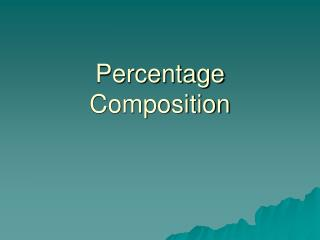DownloadDownload PresentationPercentage Composition

# Percentage Composition

Download Presentation## Percentage Composition

- - - - - - - - - - - - - - - - - - - - - - - - - - - E N D - - - - - - - - - - - - - - - - - - - - - - - - - - -
##### Presentation Transcript

1. Percentage Composition

2. What is percentage composition? The mass of each element in a compound compared to the entire mass of the compound and multiplied by 100 percent is called the percentage composition of the compound.

3. To calculate percentage composition: Mass of element X 100 = % Total mass of compound

4. The percentage composition of a compound tells you the percent of the mass made up by each element of the compound. This can be determined by calculating the molar mass of a given chemical formula.

5. Calculating percentage composition of a compound: EX: What is the percentage composition of hydrogen and oxygen in H2O? Formula Mass = H= 2 atoms X 1 amu= 2 amu O= 1 atom X 16 amu= 16 amu 18amu Percentage of Hydrogen = 2.0 g H2 18 g H2O X 100 = 11% Percentage of Oxygen = 16g O 18g H20 X 100 = 89% (CHECK: Percentages should equal 100%.)

6. Calculating mass of an element in a compound: EX: How many grams of oxygen are in 5 g of H2O? __X__ = 16g O 5 g H20 18gH20 (Cross Multiply) 18 x = (16) (5) x = 80 18 x = 4.44 g O

7. H2SO4

8. C2H5OH

9. C6H5NH2

10. Find the percentage composition of a compound that contains 2.63g of carbon, 0.370g of hydrogen, and 0.580g of oxygen in a 3.58g sample of the compound.